Saturday, December 4, 2021
Home > CBSE Class 10 > Previous Year MCQ Questions for CBSE Board Class 10 Maths with Answer Keys

# Previous Year MCQ Questions for CBSE Board Class 10 Maths with Answer Keys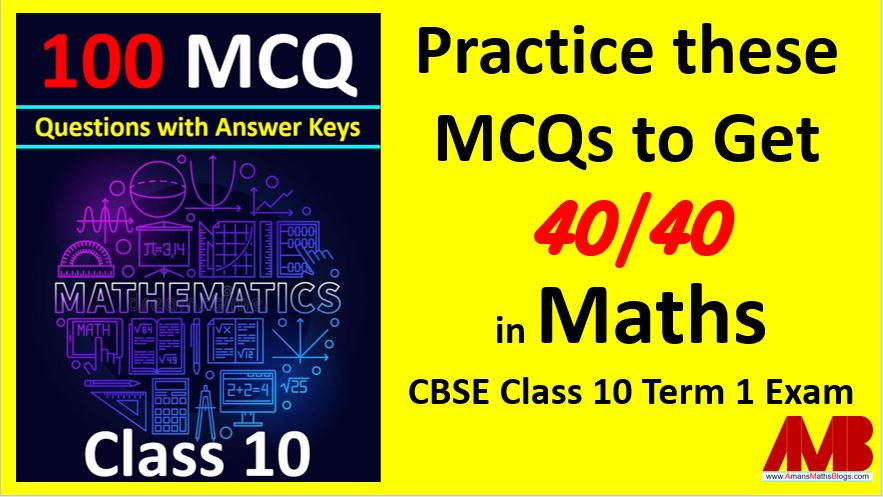## MCQ Questions for Class 10 Maths with Answer Keys

Hi students, Welcome to AMBiPi (Amans Maths Blogs). In this article, you will get Previous Year MCQ Questions for CBSE Board Class 10 Maths with Answer Keys. These multiple choice questions are taken from CBSE Board Class 10 Maths previous year questions papers and all of these questions are with the answer keys.

### MCQ Questions for Real Numbers Class 10 Maths

Real Numbers Class 10 Maths MCQ Question No 1

What is the HCF of smallest prime number and the smallest composite number ?

Option A : 1

Option B : 2

Option C : 3

Option D : 4

Option B : 2

Real Numbers Class 10 Maths MCQ Question No 2

Find LCM and HCF of 3930 and 1800.

Option A : 225800

Option B : 235600

Option C : 235800

Option D : 225600

Option B : 235800

### MCQ Questions for Polynomials Class 10 Maths

Polynomials Class 10 Maths MCQ Question No 3

If three zeroes of a polynomial x4 – x3 – 3x2 + 3x are 0, √3 and -√3, then find the fourth zero of the polynomial.

Option A : 1

Option B : -1

Option C : 2

Option D : –3

Option A : 1

Polynomials Class 10 Maths MCQ Question No 4

If the zeroes of a quadratic polynomial p(x) = ax2 + 7x + b = 0 are 2/3 and -3, then find the values of a and b.

Option A : a = 6 and b = 3

Option B : a = 3 and b = 6

Option C : a = -6 and b = 3

Option D : a = 3 and b = -6

Option D : a = 3 and b = -6

Polynomials Class 10 Maths MCQ Question No 5

Find a quadratic polynomial, the sum and product of whose zeroes are 0 and -3/5 respectively.

Option A : 3x2 – 5

Option B : x2 – 5/3

Option C : x2 – 3/5

Option D : None of these

Option C : x2 – 3/5

Polynomials Class 10 Maths MCQ Question No 6

If the quadratic polynomial p(x) = px2 – 2√5px + 15 has equal roots, then the value of p is

Option A : 0

Option B : 3

Option C : 5

Option D : 2

Option B : 3

Polynomials Class 10 Maths MCQ Question No 7

For the quadratic polynomial p(x) = 2x2 + 6√3x – 60, find the product of roots.

Option A : 30

Option B : 60

Option C : -30

Option D : -60

Option C : -30

Polynomials Class 10 Maths MCQ Question No 8

Find that non-zero value of k, for which the quadratic polynomial p(x) = (k + 1)x2 – 2(k – 1)x + 1 has equal roots.

Option A : 1

Option B : 3

Option C : 2

Option D : 4

Option B : 3

### MCQ Questions for Linear Equations Class 10 Maths

Linear Equations Class 10 Maths MCQ Question No 9

In the figure below, ABCD is a rectangle. Find the values of the product xy.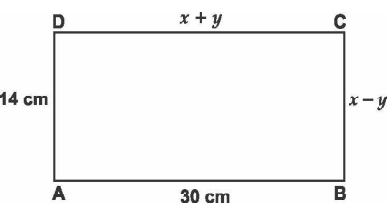Option A : 120

Option B : 254

Option C : 176

Option D : 258

Option C : 176

Linear Equations Class 10 Maths MCQ Question No 10

The system of linear equations 3x + 2y = 8 and 6x – 4y = 9 are

Option A : consistent

Option B : inconsistent

Option C : non solvable

Option D : None of these

Option A : consistent

Linear Equations Class 10 Maths MCQ Question No 11

Find the value of x + y, if 3x – y = 7 and 2x + 5y + 1 = 0.

Option A : 0

Option B : 2

Option C : 1

Option D : 3

Option C : 1

Linear Equations Class 10 Maths MCQ Question No 12

The sum of the digits of a two digit number is 8 and the difference between the number and that formed by reversing the digits is 18. Find the number.

Option A : 53

Option B : 26

Option C : 35

Option D : 62

Option A : 53

### MCQ Questions for Quadratic Equations Class 10 Maths

Quadratic Equations Class 10 Maths MCQ Question No 13

If x = 3 is one root of the quadratic equation x2 – 2kx – 6 = 0, then find the value of k.

Option A : 1/2

Option B : 3

Option C : 1/3

Option D : 2/3

Option A : 1/2

Quadratic Equations Class 10 Maths MCQ Question No 14

Find the value of p, for which one root of the quadratic equation px2 – 14x + 8 = 0 is 6 times the other.

Option A : 1/2

Option B : 3

Option C : 4

Option D : 2/5

Option B : 3

Quadratic Equations Class 10 Maths MCQ Question No 15

Find the value of k for which the equation x2 + k(2x + k – 1) + 2 = 0 has real and equal roots.

Option A : 2

Option B : 4

Option C : 6

Option D : 8

Option A : 2

Quadratic Equations Class 10 Maths MCQ Question No 16

If – 5 is a root of the quadratic equation 2x2 + px – 15 = 0 and the quadratic equation p(x2 + x) + k = 0 has equal roots, find the value of k.

Option A : 7/4

Option B : 7

Option C : 6/5

Option D : 8

Option A : 7/4

Quadratic Equations Class 10 Maths MCQ Question No 17

Find x: √(2x + 9) + x = 13

Option A : 20

Option B : 14

Option C : 8

Option D : 2

Option A : 8

Quadratic Equations Class 10 Maths MCQ Question No 18

If the roots of the quadratic equation (a – b)x2 + (b – c)x + (c – a) = 0 are equal, then

Option A : 2b = a + c

Option B : 2a = b + c

Option C : 2c = a + b

Option D : None of these

Option A : 2a = b + c

Quadratic Equations Class 10 Maths MCQ Question No 19

Three consecutive natural numbers are such that the square of the middle number exceeds the difference of the squares of the other two by 60. Find the middle number.

Option A : 9

Option B : 10

Option C : 11

Option D : 12

Option B : 10

Quadratic Equations Class 10 Maths MCQ Question No 20

If 4x2 + 4bx – (a2 – b2) = 0, then find x.

Option A : (a + b)

Option B : (a – b)

Option C : (a + b)/2

Option D : (a – b)/2

Option D : (a – b)/2

Quadratic Equations Class 10 Maths MCQ Question No 21

If x = -1/2, is a solution of the quadratic equation 3×2 + 2kx – 3= 0, find the value of k.

Option A : -9/4

Option B : 9/4

Option C : 3/5

Option D : -3/5

Option A : -9/4

### MCQ Questions for Arithmetic Progression Class 10 Maths

Arithmetic Progression Class 10 Maths MCQ Question No 22

In an AP, if the common difference (d) = –4 and the seventh term (a7) is 4, then find the first term.

Option A : 26

Option B : 25

Option C : 28

Option D : 27

Option C : 28

Arithmetic Progression Class 10 Maths MCQ Question No 23

Find the sum of first 8 multiples of 3

Option A : 102

Option B : 124

Option C : 108

Option D : 139

Option C : 108

Arithmetic Progression Class 10 Maths MCQ Question No 24

What is the common difference of an A.P. in which a21 – a7 = 84?

Option A : 3

Option B : 4

Option C : 5

Option D : 6

Option D : 6

Arithmetic Progression Class 10 Maths MCQ Question No 25

Which term of the progression 20, 19 1/4, 18 1/2, 17 3/4, … is the first negative term?

Option A : 27

Option B : 26

Option C : 28

Option D : 29

Option C : 28

Arithmetic Progression Class 10 Maths MCQ Question No 26

Which term of the A.P. 8, 14, 20, 26, … will be 72 more than its 41st term ?

Option A : 54th

Option B : 55th

Option C : 53rd

Option D : 52nd

Option C : 53rd

Arithmetic Progression Class 10 Maths MCQ Question No 27

If the 10th term of an A.P. is 52 and the 17th term is 20 more than the 13th term, find the first term.

Option A : 5

Option B : 7

Option C : 6

Option D : 4

Option A : 7

Arithmetic Progression Class 10 Maths MCQ Question No 28

Find how many integers between 200 and 500 are divisible by 8 ?

Option A : 35

Option B : 37

Option C : 36

Option D : 34

Option B : 37

Arithmetic Progression Class 10 Maths MCQ Question No 29

For the A.P. 8, 10, 12…., if it has a total of 60 terms, then find the sum of its last 10 terms.

Option A : 2536

Option B : 3726

Option C : 2850

Option D : 1170

Option D : 1170

### MCQ Questions for Triangles Class 10 Maths

Triangles Class 10 Maths MCQ Question No 30

Given ∆ABC ~ ∆PQR, if AB/PQ = 1/3, then find the ratio ar(∆ABC) / ar(∆PQR).

Option A : 1/9

Option B : 1/3

Option C : 9

Option D : 3

Option A : 1/9

Triangles Class 10 Maths MCQ Question No 31

In triangle ABC, D and E are points AC and BC respectively such that DE || AB. If AD = 2x, BE = 2x – 1, CD = x + 1 and CE = x – 1, then find the value of x.

Option A : 1/2

Option B : 1/3

Option C : 2/5

Option D : 3/7

Option B : 1/3

Triangles Class 10 Maths MCQ Question No 32

The perimeter of a right triangle is 60 cm. Its hypotenuse is 25 cm. Find the area of the triangle.

Option A : 120 cm2

Option B : 150 cm2

Option C : 180 cm2

Option D : 100 cm2

Option B : 150 cm2

Triangles Class 10 Maths MCQ Question No 33

In ∆ DEW, AB || EW. If AD = 4 cm, DE = 12 cm and DW = 24 cm, then find the value of DB.

Option A : 10

Option B : 6

Option C : 12

Option D : 8

Option D : 8

Triangles Class 10 Maths MCQ Question No 34

In given figure below, EB ⊥ AC, BG ⊥ AE and CF ⊥ AE. Which of the following is NOT true?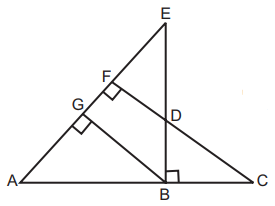Option A : ∆ABG ~ ∆DCB

Option B : ∆ABG ~ ∆ACF

Option C : ∆ABE ~ ∆AFC

Option D : None of these

Option D : None of these. All are correct.

Triangles Class 10 Maths MCQ Question No 35

In given figure below, AD = 3 cm, AE = 5 cm, BD = 4 cm, CE = 4 cm, CF = 2 cm, BF = 2.5 cm, then find the length of EF.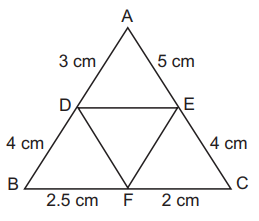Option A : 7/2

Option B : 28/9

Option C : 9/2

Option D : 25/3

Option B : 28/9

### MCQ Questions for Coordinate Geometry Class 10 Maths

Coordinate Geometry Class 10 Maths MCQ Question No 36

Find the distance of a point P(x,y) from the origin.

Option A : 0

Option B : √(x + y)

Option C : √(x2 + y2)

Option D : √(x2 – y2)

Option D : √(x2 – y2)

Coordinate Geometry Class 10 Maths MCQ Question No 37

Find the ratio in which P (4, m) divides the line segment joining the points A (2, 3) and B (6, –3). Hence find m.

Option A : Ratio = 1 : 2 and m = 0

Option B : Ratio = 1 : 3 and m = 1

Option C : Ratio = 1 : 1 and m = 0

Option D : Ratio = 1 : 4 and m = 2

Option C : Ratio = 1 : 1 and m = 0

Coordinate Geometry Class 10 Maths MCQ Question No 38

If A (–2, 1), B (a, 0), C (4, b) and D (1, 2) are the vertices of a parallelogram ABCD, find the value of (a + b).

Option A : 3

Option B : 1

Option C : 4

Option D : 2

Option D : 2

Coordinate Geometry Class 10 Maths MCQ Question No 39

A line intersects the y-axis and x-axis at the points P and Q respectively. If (2, – 5) is the mid-point of PQ, then find the co-ordinates of P and Q.

Option A : P(0, 10) and Q(4, 0)

Option B : P(0, -10) and Q(4, 0)

Option C : P(10, 0) and Q(0, 4)

Option D : P(-10, 0) and Q(0, 4)

Option B : P(0, -10) and Q(4, 0)

Coordinate Geometry Class 10 Maths MCQ Question No 40

If the distances of P(x, y), from A(5, 1) and B(– 1, 5) are equal, then

Option A : x = y

Option B : 3x = y

Option C : x = 2y

Option D : 3x = 2y

Option D : 3x = 2y

Coordinate Geometry Class 10 Maths MCQ Question No 41

If the distance between the points (4, k) and (1, 0) is 5, then what can be the possible values of k ?

Option A : k = 4

Option B : k = -5

Option C : k = 5 or -5

Option D : k = 4 or -4

Option D : k = 4 or -4

Coordinate Geometry Class 10 Maths MCQ Question No 42

Let P and Q be the points of trisection of the line segment joining the points A(2, – 2) and B(– 7, 4) such that P is nearer to A. Find the coordinates of P and Q.

Option A : P(1, 0) and Q(-4, 2)

Option B : P(-4, 2) and Q(1, 0)

Option C : P(-4, 2) and Q(-1, 0)

Option D : P(-1, 0) and Q(-4, 2)

Option D : P(-1, 0) and Q(-4, 2)

Coordinate Geometry Class 10 Maths MCQ Question No 43

If the point P(x, y) is equidistant from the points A(a + b, b – a) and B(a – b, a + b). Then,

Option A : ax = by

Option B : bx = ay

Option C : ab = xy

Option D : None of these

Option B : bx = ay

Coordinate Geometry Class 10 Maths MCQ Question No 44

The relation between x and y if the points A(x, y), B(– 5, 7) and C(– 4, 5) are collinear.

Option A : y = 2x + 3

Option B : y = 2x – 3

Option C : y = -2x + 3

Option D : y = -2x – 3

Option D : y = – 2x – 3

Coordinate Geometry Class 10 Maths MCQ Question No 45

Find the values of k so that the area of the triangle with vertices (k + 1, 1), (4, – 3) and (7, – k) is 6 sq. units.

Option A : 3

Option B : 4

Option C : -2

Option D : -1

Option A : 3

Coordinate Geometry Class 10 Maths MCQ Question No 46

Find the coordinates of a point P on the line segment joining A(1, 2) and B(6, 7) such that AP = 2AB/5.

Option A : (4, 3)

Option B : (2, 4)

Option C : (3, 4)

Option D : (4, 2)

Option C : (3, 4)

Coordinate Geometry Class 10 Maths MCQ Question No 47

If A (5, 2), B(2, – 2) and C(– 2, t) are the vertices of a right angled triangle with ∠B = 90°. then find  the value of t.

Option A : -1

Option B : 1

Option C : 1/2

Option D : 1/3

Option B : 1

### MCQ Questions for Trigonometry Class 10 Maths

Trigonometry Class 10 Maths MCQ Question No 48

What is the value of (cos267° – sin223°) ?

Option A : 0

Option B : 1

Option C : 1/2

Option D : 1/3

Option A : 0

Trigonometry Class 10 Maths MCQ Question No 49

If tan 2A = cot (A – 18°), where 2A is an acute angle, find the value of A.

Option A : 36o

Option B : 72o

Option C : 18o

Option D : 54o

Option A : 36o

Trigonometry Class 10 Maths MCQ Question No 50

In A, B and C are interior angles of triangle ABC, then sin[(A + C) / 2] is equal to

Option A : sin B/2

Option B : tan B/2

Option C : cos B/2

Option D : sec B/2

Option C : cos A/2

Trigonometry Class 10 Maths MCQ Question No 51

If x = 3 sin θ and y = 4 cos θ, find the value of √(16x2 + 9y2)

Option A : 10

Option B : 25

Option C : 13

Option D : 12

Option D : 12

Trigonometry Class 10 Maths MCQ Question No 52

In the ∆ABC, ∠A = right angle, AB = √x and BC = √(x + 5). Evaluate sinC.cosC.tanC + cos2C.sin A

Option A : 1

Option B : 0

Option C : 2

Option D : -1

Option A : 1

Trigonometry Class 10 Maths MCQ Question No 53

If ∆ ABC is right angled at B, what is the value of sin (A + C).

Option A : 0

Option B : 1

Option C : -1

Option D : 1/2

Option A : 0

Trigonometry Class 10 Maths MCQ Question No 54

If √3sinθ = cosθ, then find the value of (3cos2θ + 2cosθ) / (3cosθ + 2)

Option A : √3

Option B : 1/2

Option C : 1

Option D : √3/2

Option D : √3/2

### MCQ Questions for Application of Trigonometry Class 10 Maths

Application of Trigonometry Class 10 Maths MCQ Question No 55

If a tower 30 m high, casts a shadow 10√3 m long on the ground, then what is the angle of elevation
of the sun?

Option A : 30o

Option B : 45o

Option C : 60o

Option D : 75o

Option C : 60o

Application of Trigonometry Class 10 Maths MCQ Question No 56

From the top of a 7 m high building, the angle of elevation of the top of a tower is 60° and the angle
of depression of its foot is 45°. Find the height of the tower?

Option A : 14

Option B : 7√3

Option C : 7(√3 + 1)

Option D : 7(√3 + 2)

Option C : 7(√3 + 1)

Application of Trigonometry Class 10 Maths MCQ Question No 57

The angle of elevation of the top of a hill at the foot of a tower is 60° and the angle of elevation of the top of the tower from the foot of the hill is 30°. If height of the tower is 50 m, find the height of the hill.

Option A : 150 m

Option B : 120 m

Option C : 140 m

Option D : 130 m

Option A : 150 m

Application of Trigonometry Class 10 Maths MCQ Question No 58

A ladder, leaning against a wall, makes an angle of 60° with the horizontal. If the foot of the ladder
is 2.5 m away from the wall, find the length of the ladder.

Option A : 2.5 m

Option B : 2 m

Option C : 3.5 m

Option D : 5 m

Option D : 5 m

Application of Trigonometry Class 10 Maths MCQ Question No 59

A man standing on the deck of a ship, which is 10 m above water level, observes the angle of elevation of the top of a hill as 60° and the angle of depression of the base of hill as 30°. Find the height of the hill

Option A : 40 m

Option B : 30 m

Option C : 20 m

Option D : 15 m

Option A : 40 m

Application of Trigonometry Class 10 Maths MCQ Question No 60

As observed from the top of a light house, 100 m high above sea level, the angles of depression of a ship, sailing directly towards it, changes from 30° to 60°. Find the distance travelled by the ship during the period of observation.

Option A : 200 / √3 m

Option B : 200√3 m

Option C : 100 m

Option D : 100 / √3 m

Option A : 200 / √3 m

Application of Trigonometry Class 10 Maths MCQ Question No 61

In the figure below, a tower AB is 20 m high and BC, its shadow on the ground, is 20√3 m long. Find the angle θ.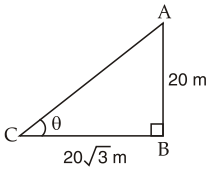Option A : 200 / √3 m

Option B : 200√3 m

Option C : 100 m

Option D : 100 / √3 m

Option A : 200 / √3 m

Application of Trigonometry Class 10 Maths MCQ Question No 62

The tops of two towers of height x and y, standing on level ground, subtend angles of 30° and 60° respectively at the centre of the line joining their feet, then find x : y.

Option A : 1 : 1

Option B : 1 : 3

Option C : 3 : 1

Option D : 1 : √3

Option B : 1 : 3

### MCQ Questions for Circles Class 10 Maths

Circles Class 10 Maths MCQ Question No 63

If the angle between two tangents drawn from an external point P to a circle of radius a and centre O, is 60°, then find the length of OP.

Option A : 3a

Option B : a

Option C : 4a

Option D : 2a

Option D : 2a

Circles Class 10 Maths MCQ Question No 64

In the given figure, PA and PB are tangents to the circle from an external point P. CD is another
tangent touching the circle at Q. If PA = 12 cm, QC = QD = 3 cm, then find PC + PD.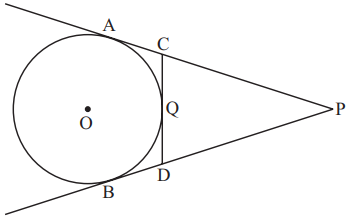Option A : 18

Option B : 15

Option C : 9

Option D : 6

Option A : 18

Circles Class 10 Maths MCQ Question No 65

In the figure below, PQ is a tangent at a point C to a circle with centre O. If AB is a diameter and ∠CAB = 30°, find ∠PCA.Option A : 30°

Option B : 45°

Option C : 60°

Option D : 75°

Option C : 60°

Circles Class 10 Maths MCQ Question No 66

In the figure below, If the radius of the circle is r and OP = 2r, then find ∠OTS.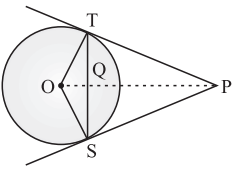Option A : 30°

Option B : 45°

Option C : 60°

Option D : 75°

Option A : 30°

Circles Class 10 Maths MCQ Question No 67

From an external point P, tangents PA and PB are drawn to a circle with centre O. If ∠ PAB = 50°, then find ∠ AOB.

Option A : 50°

Option B : 80°

Option C : 100°

Option D : 120°

Option C : 100°

Circles Class 10 Maths MCQ Question No 68

In the figure below, AP and BP are tangents to a circle with centre O, such that AP = 5 cm and ∠ APB = 60°. Find the length of chord AB.Option A : 5/2

Option B : 5√3

Option C : 5

Option D : 5/√3

Option C : 5

Circles Class 10 Maths MCQ Question No 69

In the figure below, the area of triangle ABC is 54 cm2, then find the length of AB.Option A : 15

Option B : 9

Option C : 12

Option D : 18

Option B : 9

Circles Class 10 Maths MCQ Question No 70

In the figure below, AB is the diameter of a circle with centre O and AT is a tangent. If ∠AOQ = 58°, find ∠ATQ.Option A : 29

Option B : 58

Option C : 32

Option D : 61

Option D : 61

Circles Class 10 Maths MCQ Question No 71

In the figure below, tangents PQ and PR are drawn from an external point P to a circle with centre O, such that ∠RPQ = 30°. A chord RS is drawn parallel to the tangent PQ. Find ∠RQS.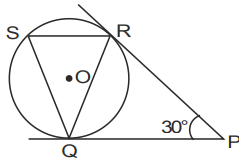Option A : 75

Option B : 60

Option C : 30

Option D : 50

Option C : 30

### MCQ Questions for Area Related to Circles Class 10 Maths

Area Related to Circles Class 10 Maths MCQ Question No 72

In the given figure below, ABCD is a rectangle of dimensions 21 cm × 14 cm. A semicircle is drawn
with BC as diameter. Find the area of the shaded region in the figure.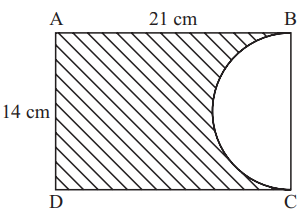Option A : 217 cm2

Option B : 249 cm2

Option C : 235 cm2

Option D : 223 cm2

Option A : 217 cm2

Area Related to Circles Class 10 Maths MCQ Question No 73

In the given figure below, ∆ ABC is a right-angled triangle in which ∠ A is 90°. Semicircles are drawn on AB, AC and BC as diameters. Find the area of the shaded region.Option A : 7 cm2

Option B : 4 cm2

Option C : 5 cm2

Option D : 6 cm2

Option D : 6 cm2

Area Related to Circles Class 10 Maths MCQ Question No 74

In the given figure below, OACB is a quadrant of a circle with centre O and radius 3.5 cm. If OD = 2 cm, find the area of the shaded region.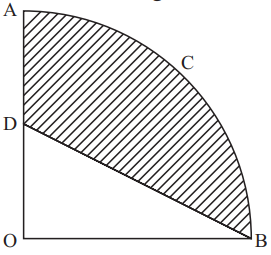Option A : 49 cm2

Option B : 49/8 cm2

Option C : 49/6 cm2

Option D : 49/2 cm2

Option B : 49/8 cm2

Area Related to Circles Class 10 Maths MCQ Question No 75

In the given figure below, O is the centre of a circle such that diameter AB = 13 cm and AC = 12 cm. BC is joined. Find the area of the shaded region.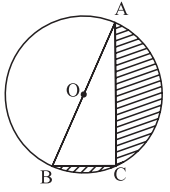Option A : 30 cm2

Option B : 66.33 cm2

Option C : 36.33 cm2

Option D : 46 cm2

Option C : 36.33 cm2

Area Related to Circles Class 10 Maths MCQ Question No 76

Find the area of the minor segment of a circle of radius 14 cm, when its central angle is 60°.

Option A : 17.89 cm2

Option B : 18.36 cm2

Option C : 15.52 cm2

Option D : 14.39 cm2

Option A : 17.89 cm2

Area Related to Circles Class 10 Maths MCQ Question No 77

All the vertices of a rhombus lie on a circle. Find the area of the rhombus, if the area of the circle is 400π cm2.

Option A : 400 cm2

Option B : 600 cm2

Option C : 800 cm2

Option D : 200 cm2

Option C : 800 cm2

Area Related to Circles Class 10 Maths MCQ Question No 78

The diagonal of a rectangular field is 16 m more than the shorter side. If the longer side is 14 m more than the shorter side, then find the lengths of the rectangular field.

Option A : 24 cm

Option B : 12 cm

Option C : 18 cm

Option D : 26 cm

Option A : 24 cm

### MCQ Questions for Surface Area and Volumes Class 10 Maths

Surface Area & Volumes Class 10 Maths MCQ Question No 79

The slant height of a frustum of a cone is 4 cm and the perimeters of its circular ends are 18 cm and
6 cm. Find the curved surface area of the frustum.

Option A : 35 cm2

Option B : 48 cm2

Option C : 65 cm2

Option D : 54 cm2

Option B : 48 cm2

Surface Area & Volumes Class 10 Maths MCQ Question No 80

Volume and surface area of a solid hemisphere are numerically equal. What is the diameter of hemisphere ?

Option A : 8

Option B : 7

Option C : 6

Option D : 9

Option D : 9

Surface Area & Volumes Class 10 Maths MCQ Question No 81

A metallic solid sphere of radius 10.5 cm is melted and recasted into smaller solid cones, each of radius 3.5 cm and height 3 cm. How many cones will be made ?

Option A : 125

Option B : 124

Option C : 127

Option D : 126

Option D : 126

Surface Area & Volumes Class 10 Maths MCQ Question No 82

A solid metallic sphere of diameter 16 cm is melted and recasted into smaller solid cones, each of radius 4 cm and height 8 cm. Find the number of cones so formed.

Option A : 16

Option B : 14

Option C : 17

Option D : 15

Option A : 16

Surface Area & Volumes Class 10 Maths MCQ Question No 83

A well of diameter 4 m is dug 21 m deep. The earth taken out of it has been spread evenly all around it in the shape of a circular ring of width 3 m to form an embankment. Find the height of the embankment.

Option A : 4 m

Option B : 6 m

Option C : 8 m

Option D : 9 m

Option A : 4 m

Surface Area & Volumes Class 10 Maths MCQ Question No 84

The sum of the radius of base and height of a solid right circular cylinder is 37 cm. If the total surface area of the solid cylinder is 1628 sq. cm, find the volume of the cylinder.

Option A : 3430 m3

Option B : 4200 m3

Option C : 4620 m3

Option D : 3260 m3

Option C : 4620 m3

Surface Area & Volumes Class 10 Maths MCQ Question No 85

The length, breadth and height of a room are 8 m 50 cm, 6 m 25 cm and 4 m 75 cm respectively. Find the length of the longest rod that can measure the dimensions of the room exactly.

Option A : 25 cm

Option B : 50 cm

Option C : 75 cm

Option D : 100 cm

Option A : 25 cm

### MCQ Questions for Statistics Class 10 Maths

Statistics Class 10 Maths MCQ Question No 86

If empirical relationship between mean, median and mode is expressed as mean = k(3 median – mode), then find the value of k

Option A : 1/2

Option B : 1/7

Option C : 2/9

Option D : 5/9

Option A : 1/2

Statistics Class 10 Maths MCQ Question No 87

For the following distribution, find the median class.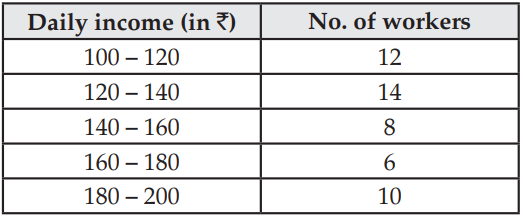Option A : 140-160

Option B : 180-200

Option C : 160-180

Option D : 120-140

Option D : 120-140

Statistics Class 10 Maths MCQ Question No 88

From the following frequency distribution, find the median class :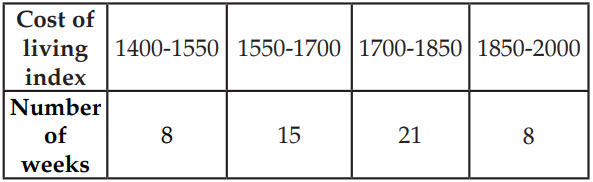Option A : 1400-1550

Option B : 1550-1700

Option C : 1700-1850

Option D : 1850-2000

Option A : 1/2

### MCQ Questions for Probability Class 10 Maths

Probability Class 10 Maths MCQ Question No 89

An integer is chosen at random between 1 and 100. Find the probability that it is :

Option A : 5/49

Option B : 6/49

Option C : 8/49

Option D : 9/49

Option B : 6/49

Probability Class 10 Maths MCQ Question No 90

A bag contains 15 white and some black balls. If the probability of drawing a black ball from the bag is thrice that of drawing a white ball, find the number of black balls in the bag

Option A : 15

Option B : 30

Option C : 45

Option D : 60

Option C : 45

Probability Class 10 Maths MCQ Question No 91

Two different dice are thrown together. Find the probability that the numbers obtained is a doublet of odd numbers.

Option A : 5/12

Option B : 1/12

Option C : 1/3

Option D : 1/4

Option B : 1/12

Probability Class 10 Maths MCQ Question No 92

A card is drawn at random from a well shuffled pack of 52 playing cards. Find the probability of getting neither a red card nor a queen.

Option A : 5/13

Option B : 6/13

Option C : 7/26

Option D : 3/52

Option B : 6/13

Probability Class 10 Maths MCQ Question No 93

Three different coins are tossed together. Find the probability of getting at least two heads.

Option A : 3/8

Option B : 1/4

Option C : 1/2

Option D : 1/8

Option B : 1/4

Probability Class 10 Maths MCQ Question No 94

A number x is selected at random from the numbers 1, 2, 3 and 4. Another number y is selected at random from the numbers 1, 4, 9 and 16. Find the probability that product of x and y is less than 16.

Option A : 1/2

Option B : 1/8

Option C : 1/4

Option D : 5/16

Option A : 1/2

Probability Class 10 Maths MCQ Question No 95

From a pack of 52 playing cards, Jacks, Queens and Kings of red colour are removed. From the remaining, a card is drawn at random. Find the probability that drawn card is a card of red colour.

Option A : 1/23

Option B : 10/23

Option C : 1/13

Option D : 10/13

Option B : 10/23

Probability Class 10 Maths MCQ Question No 96

Cards marked with number 3, 4, 5, ….., 50 are placed in a box and mixed thoroughly. A card is drawn at random from the box. Find the probability that the selected card bears a perfect square number.

Option A : 1/8

Option B : 1/6

Option C : 3/16

Option D : 5/16

Option A : 1/8

Probability Class 10 Maths MCQ Question No 97

A card is drawn at random from a well-shuffled deck of playing cards. Find the probability that the card drawn is either a king or a queen.

Option A : 11/13

Option B : 2/13

Option C : 3/13

Option D : 5/13

Option B : 2/13

Probability Class 10 Maths MCQ Question No 98

A bag contains white, black and red balls only. A ball is drawn at random from the bag. If the probability of getting a white ball is 3/10 and of a black ball is 2/5, then find the probability of getting a red ball.

Option A : 7/10

Option B : 3/5

Option C : 3/10

Option D : 5/17

Option C : 3/10

Probability Class 10 Maths MCQ Question No 99

A letter of English alphabet is chosen at random. Determine the probability that the chosen letter is a consonant.

Option A : 4/13

Option B : 1/13

Option C : 5/26

Option D : 21/26

Option D : 21/26

Probability Class 10 Maths MCQ Question No 100

A box contains 20 cards numbered from 1 to 20. A card is drawn at random from the box. Find the probability that the number on the drawn card is divisible by 2 or 3.

Option A : 1/4

Option B : 1/5

Option C : 11/20

Option D : 13/20

Option D : 13/20

CBSE Class 10 Maths Chapterwise MCQs
1 : Real Numbers MCQ
| 1 to 10 | 11 to 20 | 21 to 30 | 31 to 40 | 41 to 50
2 : Polynomials MCQ
| 1 to 10 | 11 to 20 | 21 to 30 | 31 to 40 | 41 to 50
3 : Linear Equations MCQ
| 1 to 10 | 11 to 20 | 21 to 30 | 31 to 40 | 41 to 50
| 1 to 10 | 11 to 20 | 21 to 30 | 31 to 40 | 41 to 50
5 : Arithmetic Progression MCQ
| 1 to 10 | 11 to 20 | 21 to 30 | 31 to 40 | 41 to 50
6 : Triangles MCQ
| 1 to 10 | 11 to 20 | 21 to 30 | 31 to 40 | 41 to 50
7 : Coordinate Geometry MCQ
| 1 to 10 | 11 to 20 | 21 to 30 | 31 to 40 | 41 to 50
8 : Introduction to Trigonometry MCQ
| 1 to 10 | 11 to 20 | 21 to 30 | 31 to 40 | 41 to 50
9 : Application of Trigonometry MCQ
| 1 to 10 | 11 to 20 | 21 to 30 | 31 to 40 | 41 to 50
10 : Circles MCQ
| 1 to 10 | 11 to 20 | 21 to 30 | 31 to 40 | 41 to 50
11 : Constructions MCQ
| 1 to 10 | 11 to 20
12 : Area Related to Circles MCQ
| 1 to 10 | 11 to 20 | 21 to 30 | 31 to 40 | 41 to 50
13 : Surface Area & Volume MCQ
| 1 to 10 | 11 to 20 | 21 to 30 | 31 to 40 | 41 to 50
14 : Statistics MCQ
| 1 to 10 | 11 to 20 | 21 to 30 | 31 to 40 | 41 to 50
15 : Probability MCQ
| 1 to 10 | 11 to 20 | 21 to 30 | 31 to 40 | 41 to 50
16 : Previous Year Maths MCQs from CBSE Board Class 10
| 1 to 100
AMBiPi
error: Content is protected !!